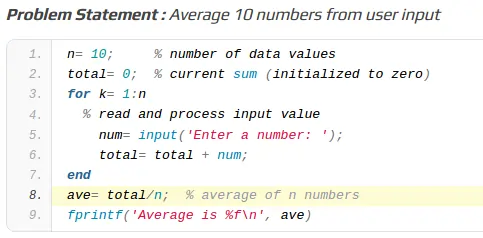# MATLAB Assignment Samples – Find The Average Value`MATLAB Assignment Samples –`

`Problem Statement : A stick of unit length is randomly split in two pieces. Estimate the average length of the short piece.`

``````n= 10000;  % number of trials
total= 0;  % accumulated length of short pieces so far

for k= 1:n
breakPt= rand;
shortPiece= min(breakPt, 1-breakPt);
total= total + shortPiece;
end

aveLength= total/n;
fprintf('Estimated average length is %.4f (%d trials)
', ...
aveLength, n)``````

`Problem Statement: Show screen output for the stick experiment in order to illustrate the for loop of the above assignment`

``````clc
n= 10;     % number of trials
total= 0;  % accumulated length of short pieces so far

for k= 1:n
fprintf('Top of loop.  k is %d. ', k)
breakPt= rand;
fprintf(' Split at %.2f. ', breakPt)
shortPiece= min(breakPt, 1-breakPt);
total= total + shortPiece;
fprintf(' Bottom of loop.
')
pause
end

aveLength= total/n;
fprintf('Estimated average length is %.4f (%d trials)
',...
aveLength, n)``````

`Problem Statement : Average 10 numbers from user input`

``````n= 10;     % number of data values
total= 0;  % current sum (initialized to zero)
for k= 1:n
% read and process input value
num= input('Enter a number: ');
total= total + num;
end
ave= total/n;  % average of n numbers
fprintf('Average is %f
', ave)``````

`Read Also : Get Any Kind of Engineering Assignment Sample Online `

Matlab Assignment, Engineering Assignment , MATLAB Assignment Samples,